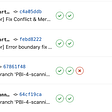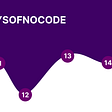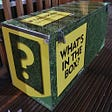# C# Challenge - Tip Calculator

Today, we have the following objective:

To get started, we need to create some variables.

We need 10 variables in total:

• One for storing the dinner bill price
• A variable for the calculated 15% tip
• A variable for the calculated 20% tip
• A variable for the calculated 25% tip
• A variable to enter a custom% tip
• A variable for the calculated custom% tip
• A variable for the total sum with a 15% tip
• A variable for the total sum with a 20% tip
• A variable for the total sum with a 25% tip
• A variable for the total sum with a custom% tip

Calculating the tip amount
To calculate the tip amount, we simply need to multiply the dinner bill by 0.<tipValue>.

As for the custom entered tip, we need to take the dinner bill and multiply it by the custom tip value divided by 100:

Calculate the total sum
To calculate the total sum, we simply need to add the calculated tip value to the dinner bill.

Printing out the total sum
To print out the calculated sum, we just need to tell the percentage of the tip and the total sum.

--

--

--

## More from Timo Schmid

The mission? Becoming a game developer! RPG is the dream! Writing down my journey here for me and for everyone interested. Thanks for showing interest :)

Love podcasts or audiobooks? Learn on the go with our new app.

## GitLab, the real time machine.## Day 8–15 of My Nocode Challenge## 5 Powerful Ways To Use Artificial Intelligence In eCommerce## An Intro to Features## Recap: The 16th Class of RARA NFT School## Setup Vuforia with Unity## Caveats of using return with try/except in Python## Timo Schmid

The mission? Becoming a game developer! RPG is the dream! Writing down my journey here for me and for everyone interested. Thanks for showing interest :)

## How Do Hackers Hacks Windows 11 with using Android Phone in Lan Area Network ? — Security Advices